# 5 Growth Plays: 2 Excellent, 1 Good, 2 Middle Of The Road

|
Includes: AT, FMS, NLY, SHLDQ, VAR
by: Tactical Investor

"The rich get richer. Not only because they have surpluses with which to invest, but because of the overriding emotional release they experience from having wealth."

Stuart Wilde

The markets are extremely overbought, and it would be prudent for long-term investors to wait for a strong pullback before committing large sums of money to this market. Investors looking for additional streams of income can consider the following two options:

1. Investors can sell covered calls.
2. If one is bullish on a stock, one can sell naked puts and open an additional stream of income. For example, if you like CLARCOR Inc. (CLC) but only find it attractive in the 44-46 ranges, you could sell puts with a strike at 45. If the stock trades below this price on the last day, you will have to buy the stock. However, your final price will be much lower, as you would subtract the premium you received from the price you paid for the shares. On the other hand, if the stock does not close below this price, then you get to keep the premium.

Fresenius Medical Care AG & Co (NYSE:FMS) is our favorite play on this list for the following reasons:

• A strong levered free cash flow of 687 million
• Net income increased from \$965 million in 2009 to \$1.17 billion in 2011
• EBITDA increased from \$2.23 billion in 2009 to \$2.7 billion in 2011
• Total cash flow from operating activities increased from \$1.34 billion in 2009 to \$1.45 billion in 2011
• Cash flow per share increased from \$4.61 in 2009 to \$5.42 in 2011
• Annual EPS before NRI has jumped from \$2.43 in 2007 to \$3.54 in 2011
• A decent ROE of 13.07%
• A good quarterly earning's growth of 14.4%
• Decent current and quick ratios of 1.34 and 1.11, respectively
• A good interest coverage ratio of 6.08
• A five-year sales growth of 8.17%
• Cash And Cash Equivalents have increased from \$301 million in 2009 to \$457 in 2011.
• Gross profit has increased from \$3.8 billion in 2009 to \$4.5 billion in 2011
• A very low payout ratio of 19% and five year average payout ratio of only 20%
• A five-year dividend growth rate of 11.06%
• A good three year total return of 87%
• \$100K invested for 10 years would have grown to \$370K.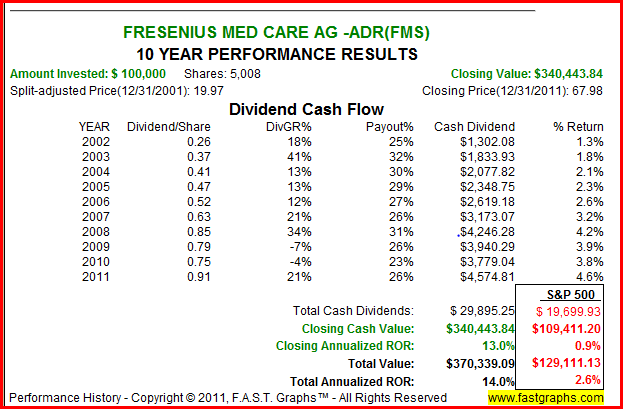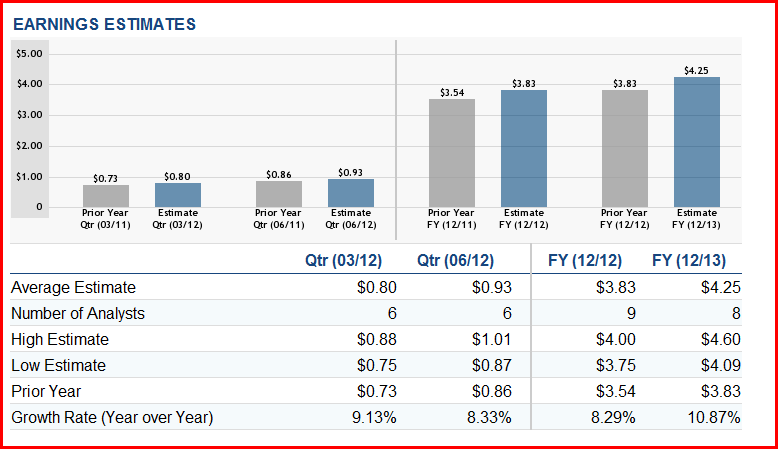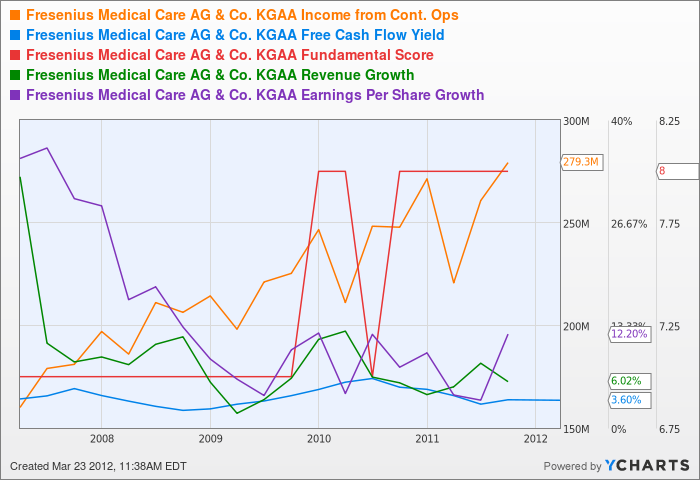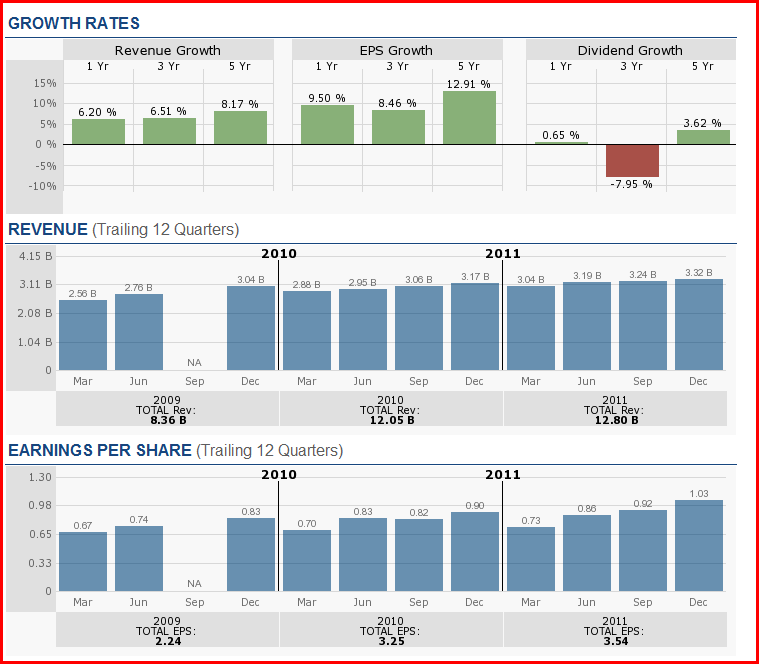As many key ratios are featured in this article, it would be in the investor's best interests to get a handle on the more important ones that are listed below. Getting a handle on these ratios could help you come up with your own system for spotting potential winners.

Long-term debt-to-equity ratio is the total long-term debt divided by the total equity. The amount of long-term debt a company carries on its balances sheet is very important, for it indicates the amount of money a company owes that it doesn't expect to pay off in the next year. A balance sheet that illustrates that long-term debt has been decreasing for a few years is a sign that the company is doing well. When debt levels fall, and cash levels increase, the balance sheet is said to be improving, and vice versa. If a company has too much debt on its books, it could end up being overwhelmed with interest payments and risk having too little working capital, which could in the worst case scenario lead to bankruptcy.

Operating cash flow is generally a better metric than earnings per share because a company can show positive net earnings and still not be able to properly service its debt; the cash flow is what pays the bills.

The payout ratio tells us what portion of the profit is being returned to investors. A pay out ratio over 100% indicates that the company is paying out more money to shareholders, than it is making; this situation cannot last forever. In general, if the company has a high operating cash flow and access to capital markets, it can keep this going on for a while. As companies usually only pay the portion of the debt that is coming due and not the whole debt, this technique/trick can technically be employed to maintain the dividend for sometime. If the payout ratio continues to increase, the situation warrants close monitoring as this cannot last forever; if your tolerance for risk is a low, look for similar companies with the same or higher yields, but with lower payout ratios. Individuals searching for other ideas might find this article to be of interest 5 Growth Plays To Consider: 2 Great, 1 Good And 2 Middling.

Current Ratio is obtained by dividing the current assets by current liabilities. This ratio allows you to see if the company can pay its current debts without potentially jeopardizing its future earnings. Ideally, the company should have a ratio of 1 or higher.

Interest coverage is usually calculated by dividing the earnings before interest and taxes for a period of 1 year by the interest expenses for the same time period. This ratio informs you of a company's ability to make its interest payments on its outstanding debt. Lower interest coverage ratios indicate that there is a larger debt burden on the company and vice versa. For example, if a company has an interest ratio of 11.8, this means that it covers interest expenses 11.8 times with operating profits.

Price to tangible book is obtained by dividing share price by tangible book value per share. The ratio gives investors some idea of whether they are paying too much for what would be left over if the company were to declare bankruptcy immediately. In general, stocks that trade at higher price to tangible book value could leave investors facing a great percentage per share loss than those that trade at lower ratios. The price to tangible book value is theoretically the lowest possible price the stock would trade to. Additional key metrics are addressed in this article 2 Good, And 3 Middle Of The Road Dividend Plays.

Fresenius Medical Care AG & Co (FMS)

Industry : Diagnostic & Health Related Services

Levered Free Cash Flow: 684.74M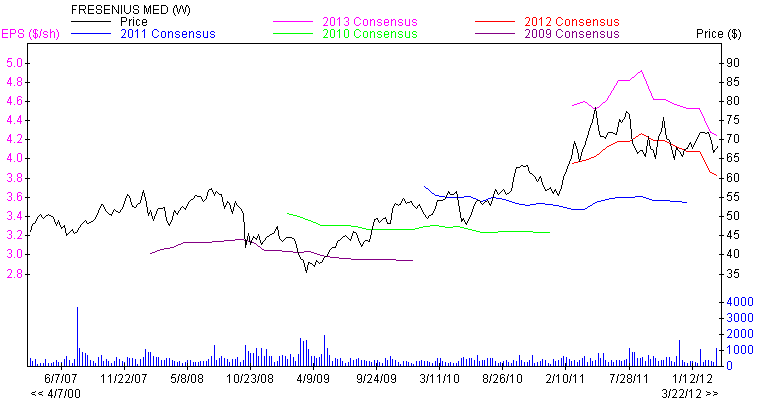Growth

Net income for the past three years:

• Net Income 2009 = \$965 million
• Net Income 2010 = \$1065 million
• Net Income 2011 = \$1177 million

EBITDA:

• EBITDA 12/2011 = \$2692 million
• EBITDA 12/2010 = \$2452 million
• EBITDA 12/2009 = \$2234 million

Net income Reported Quarterly = \$226 million

Total cash flow from operating activities:

• 2009 = \$1.34 billion
• 2010 = \$1.37 billion
• 2011 = \$1.45 billion

Cash Flow:

• Cash Flow 12/2011 = 5.42 \$/share
• Cash Flow 12/2010 = 4.97 \$/share
• Cash Flow 12/2009 = 4.61 \$/share

Annual EPS before NRI:

• Annual EPS before NRI 12/2011 = 3.54
• Annual EPS before NRI 12/2010 = 3.25
• Annual EPS before NRI 12/2009 = 2.99
• Annual EPS before NRI 12/2008 = 2.79
• Annual EPS before NRI 12/2007 = 2.43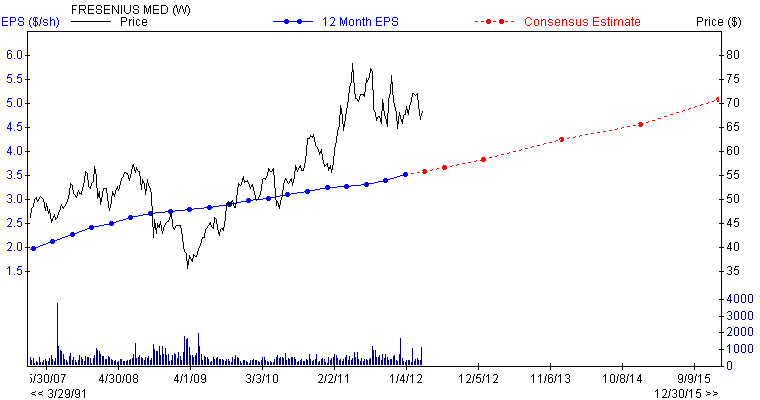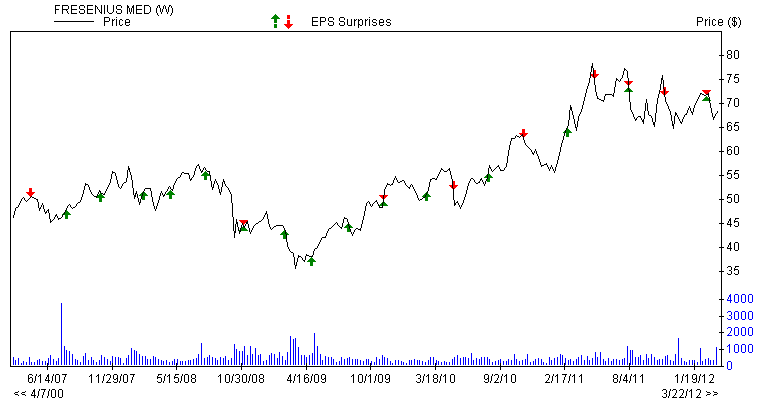Performance

• ROE = 13.07%
• Return on Assets = 5.69%
• Quarterly Earnings Growth = 14.4%
• Quarterly Revenue Growth = 4.9%
• Price to Sales = 1.6
• Price to Book = 2.41
• Price to Tangible Book = -10.33
• Price to Cash Flow = 12.54
• Price to Free Cash Flow = -15.3
• Total return last 3 years = 87.18%
• Total return last 5 years = 53.75%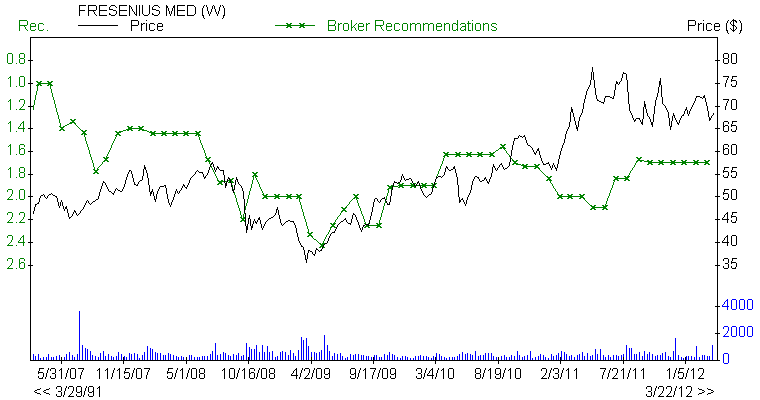Dividend history and Sustainability

• Current Ratio 09/2011 = 1.34
• Current Ratio 5 Year Average = 1.39
• Quick Ratio = 1.11
• Cash Ratio = 0.45
• Interest Coverage 09/2011 = 6.08
• Payout Ratio 09/2011 = 0.19
• Payout Ratio 06/2011 = 0.19
• Payout Ratio 5 Year Average 09/2011 = 0.2
• Payout Ratio 5 Year Average 06/2011 = 0.2
• Change in Payout Ratio = -0.02
• Dividend yield 5 year average = 1.1%
• Dividend growth rate 3 year Average = 1.26%
• Dividend growth rate 5 year average = 11.06%
• Paying dividends since = 1998

Note: This falls under the category of "excellent or first class"

Varian Medical Systems, Inc. (NYSE:VAR)

Industry : Medical Instruments & Equipment

Levered Free Cash Flow: 301.75M

Growth

Net income for the past three years:

• Net Income 2009 = \$319 million
• Net Income 2010 = \$360 million
• Net Income 2011 = \$399 million

EBITDA:

• EBITDA 12/2011 = \$644 million
• EBITDA 12/2010 = \$585 million
• EBITDA 12/2009 = \$523 million

Net income Reported Quarterly = \$90 million

Total cash flow from operating activities:

• 2009 = \$304.44 million
• 2010 = \$460.79 million
• 2011 = \$472.78 million
• Cash Flow 12/2011 = 3.94 \$/share
• Cash Flow 12/2010 = 3.42 \$/share
• Cash Flow 12/2009 = 3 \$/share

Annual EPS before NRI:

• Annual EPS before NRI 12/2011 = 3.44
• Annual EPS before NRI 12/2010 = 2.96
• Annual EPS before NRI 12/2009 = 2.65
• Annual EPS before NRI 12/2008 = 2.31
• Annual EPS before NRI 12/2007 = 1.85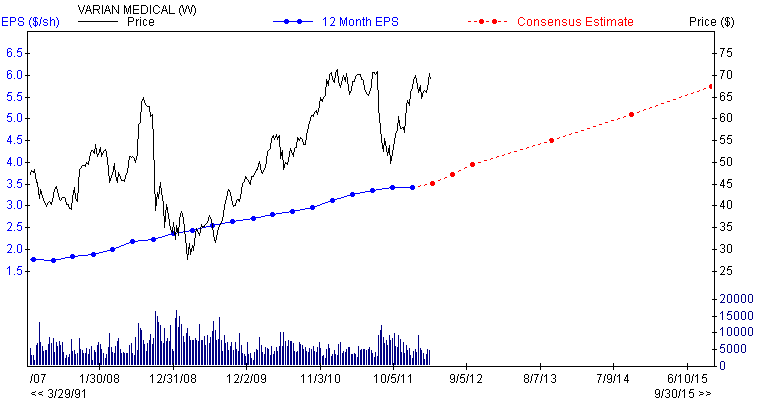Performance

• ROE = 29.95%
• Return on Assets = 15.99%
• Quarterly Earnings Growth = -6.5%
• Quarterly Revenue Growth = 7.8%
• Price to Sales = 2.96
• Price to Book = 5.73
• Price to Tangible Book = 6.82
• Price to Cash Flow = 17.58
• Price to Free Cash Flow = 25
• Current Ratio 09/2011 = 1.75
• Current Ratio 5 Year Average = 1.83
• Quick Ratio = 1.28
• Cash Ratio = 0.72
• Interest Coverage 09/2011 = 158.61
• Total return last 3 years = 140.44%
• Total return last 5 years = 43.12%

Note: This falls under the category of "excellent"

Atlantic Power Corp (NYSE:AT)

Industry : Electric Utilities

Levered Free Cash Flow : -49.21M

Growth

Net income for the past three years:

• Net Income 2009 = \$-38 million
• Net Income 2010 = \$-4 million
• Net Income 2011 = \$-35 million

EBITDA:

• EBITDA 12/2011 = \$20 million
• EBITDA 12/2010 = \$55 million
• EBITDA 12/2009 = \$-13 million
• Net income Reported Quarterly = \$226 million

Total cash flow from operating activities:

• 2009 = \$50.45 million
• 2010 = \$86.96 million
• 2011 = \$55.94 million
• Cash Flow 12/2011 = 0.32 \$/share
• Cash Flow 12/2010 = 0.55 \$/share
• Cash Flow 12/2009 = 0.05 \$/share

Annual EPS before NRI:

• Annual EPS before NRI 12/2011 = -0.4
• Annual EPS before NRI 12/2010 = -0.06
• Annual EPS before NRI 12/2009 = -0.63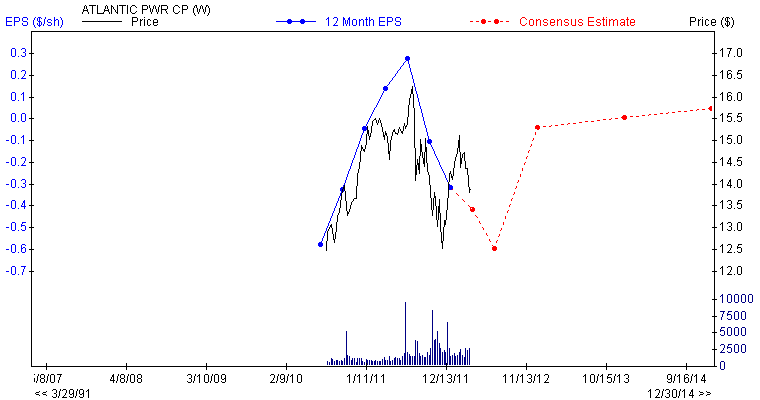Performance

• ROE = -5.06%
• Return on Assets = -1.73%
• Quarterly Revenue Growth = 172.6%
• Price to Sales = 5.5
• Price to Book = 1.75
• Price to Tangible Book = -7.24
• Price to Cash Flow = 43.06
• Price to Free Cash Flow = -10.9
• Current Ratio 09/2011 = 1.04
• Current Ratio 5 Year Average = 1.61
• Quick Ratio = 0.95
• Cash Ratio = 0.52
• Interest Coverage =0.10
• Paying dividends since = 1961

Notes: This falls under the category of "average".

Sears Holdings Corp (SHLD)

Industry: Retail - General Merchandise/Department Stores

Levered Free Cash Flow: 608.38M

Growth

Net income:

• Net Income 2010 = \$235 million
• Net Income 2011 = \$133 million

EBITDA:

1. EBITDA 12/2011 = \$1328 million
• EBITDA 12/2010 = \$1533 million
• Net income Reported Quarterly = \$226 million

Total cash flow from operating activities:

• 2009 = \$992 million
• 2010 = \$1.51 billion
• 2011 = \$130 million
• Cash Flow 12/2011 = 10 \$/share
• Cash Flow 12/2010 = 10.99 \$/share

Annual EPS before NRI:

• Annual EPS before NRI 12/2011 = 2.07
• Annual EPS before NRI 12/2010 = 3.19
• Annual EPS before NRI 12/2009 = 1.69
• Annual EPS before NRI 12/2008 = 5.32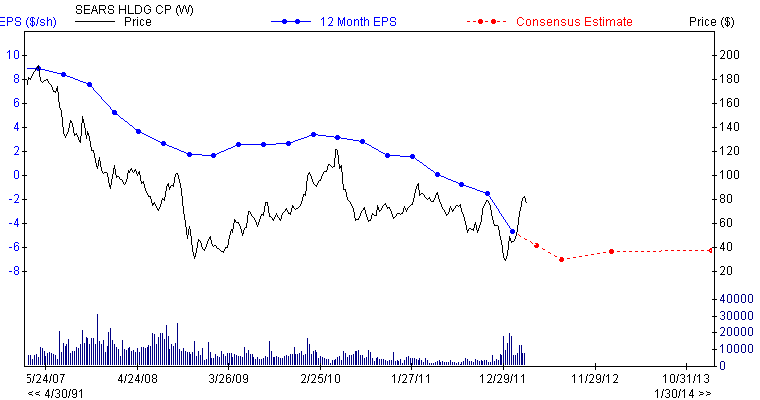Performance

• ROE = -6.99%
• Return on Assets = -2.1%
• Total return last 3 years = 116.21%
• Total return last 5 years = -52.44%
• Price to Sales = 0.21
• Price to Book = 2.03
• Price to Tangible Book = 17.46
• Price to Cash Flow = 23.78
• Price to Free Cash Flow = -12.4
• Current Ratio 03/2012 = 1.11
• Current Ratio 12/2011 = 1.11
• Current Ratio 09/2011 = 1.15
• Current Ratio 5 Year Average = 1.29
• Quick Ratio = 0.2
• Cash Ratio = 0.12

Annaly Cap Mgmt (NYSE:NLY)

Free Cash Flow = \$2.4 billion

Basic Key ratios

• Percentage Held by Insiders = 1.04
• Market Cap (\$mil) = 15912

Growth

Net Income:

• Net Income (\$mil) 12/2011 = 344
• Net Income (\$mil) 12/2010 = 1267
• Net Income (\$mil) 12/2009 = 1961
• 12months Net Income this Quarterly/ 12months Net Income 4Q's ago = -72.82
• Quarterly Net Income this Quarterly/ same Quarter year ago = -63.43
• Net Income Reported Quarterlytr (\$mil) = 446
• Annual Net Income this Yr/ Net Income last Yr = -72.82

EBITDA:

• EBITDA (\$mil) 12/2011 = 1691
• EBITDA (\$mil) 12/2010 = 2400
• EBITDA (\$mil) 12/2009 = 2828

Cash Flow:

• Cash Flow (\$/share) 12/2011 = 3.17
• Cash Flow (\$/share) 12/2010 = 3.39
• Cash Flow (\$/share) 12/2009 = 3.25

Sales:

• Sales (\$mil) 12/2011 = 1120
• Sales (\$mil) 12/2010 = 2635
• Sales (\$mil) 12/2009 = 3424

Annual EPS before NRI:

• Annual EPS before NRI 12/2007 = 1.27
• Annual EPS before NRI 12/2008 = 2.2
• Annual EPS before NRI 12/2009 = 2.76
• Annual EPS before NRI 12/2010 = 2.31
• Annual EPS before NRI 12/2011 = 2.57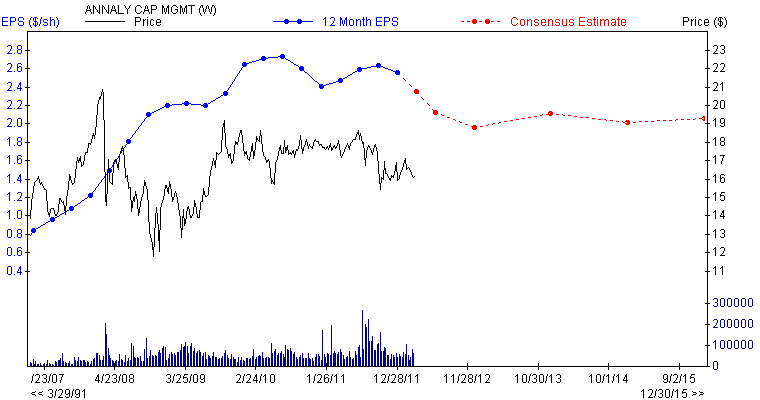Dividend history

• Dividend Yield = 13.9
• Dividend Yield 5 Year Average 12/2011 = 13.10
• Annual Dividend 12/2011 = 2.44
• Annual Dividend 12/2010 = 2.65
• Forward Yield = 13.9
• Dividend 5 year Growth 12/2011 = 70%

Dividend sustainability

• Payout Ratio 06/2011 = 0.89
• Payout Ratio 5 Year Average 06/2011 = 1.03
• Change in Payout Ratio = -0.14
• Interest coverage= 1.80

Performance

• Percentage Change Price 52 Weeks Relative to S&P 500 = -15.16
• Next 3-5 Year Estimate EPS Growth rate = 2
• EPS Growth Quarterly(1)/Q(-3) = 113.33
• ROE 5 Year Average 06/2011 = 14.7
• Return on Investment 06/2011 = 14.32
• Debt/Total Cap 5 Year Average 06/2011 = 3.05
• Current Ratio 06/2011 = 0.04
• Current Ratio 5 Year Average = 0.04
• Quick Ratio = 0.04
• Cash Ratio = 0.02

Valuation

• Book Value Quarterly = 16.06
• Price/ Book = 1.02
• Price/ Cash Flow = 5.18
• EV/EBITDA 12 Mo = 9.38

Note: This falls under the category of "good".

EPS, EPS surprise, broker recommendations, and price and consensus charts sourced from zacks.com. Earning's estimates and growth rate charts sourced from dailyfinance.com. Free cash flow yield, income from cont operations, and revenue growth sourced from Ycharts.com.

Disclosure: I have no positions in any stocks mentioned, and no plans to initiate any positions within the next 72 hours.

Additional disclosure: This list of stocks is meant to serve as a starting point. Please do not treat this as a buying list. It is imperative that you do your due diligence and then determine if any of the above plays meet with your risk tolerance levels. The Latin maxim caveat emptor applies-let the buyer beware